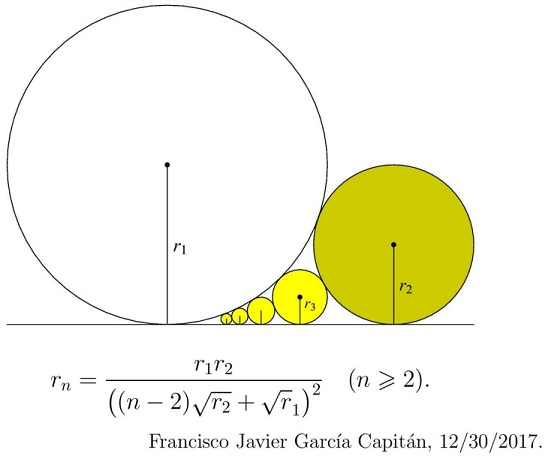# An Extension of a Sangaku with Touching Circles

### Source### Solution 1

We rewrite the problem in the form $\displaystyle \frac{1}{\sqrt{r_n}}=\frac{n-2}{\sqrt{r_1}}+\frac{1}{\sqrt{r_2}}$ and, for a proof, use the induction on the number $n$ of the circles.

For $n=3,$ this is the subject of an earlier Sangaku.

Assume the statement holds for $n=k\ge 3,$ which we'll write as $\displaystyle \frac{1}{\sqrt{r_k}}=\frac{k-2}{\sqrt{r_1}}+\frac{1}{\sqrt{r_2}}.$ Let's prove it for $n=k+1.$

From the basic step,

\displaystyle\begin{align}\frac{1}{\sqrt{r_{k+1}}}&=\frac{1}{\sqrt{r_1}}+\frac{1}{\sqrt{r_k}}\\ &=\frac{1}{\sqrt{r_1}}+\left(\frac{k-2}{\sqrt{r_1}}+\frac{1}{\sqrt{r_2}}\right)\\ &=\frac{k-1}{\sqrt{r_1}}+\frac{1}{\sqrt{r_2}}, \end{align}

which is the required identity.

### Solution 2

For two touching circles with radii $R$ and $r$ such that $R\geq r$, let the angle made by the line through the centers of the circles with the horizontal be $\phi(R,r)$. Thus,

$\displaystyle\sin\phi(R,r)=\frac{R-r}{R+r} \Rightarrow \cos\phi(R,r)=2\frac{\sqrt{rR}}{R+r}.$

Consider the three circles with radii $r_1$, $r_{n-1}$, and $r_n$. Applying the constraint for the horizontal distance between the centers,

\begin{align} (r_1+r_{n-1})\cos\phi(r_1,r_{n-1})&=(r_1+r_n)\cos\phi(r_1,r_n)+(r_{n-1}+r_n)\cos\phi(r_{n-1},r_n) \\ \Rightarrow \sqrt{r_1r_{n-1}}&=\sqrt{r_1r_n}+\sqrt{r_{n-1}r_n} \\ \Rightarrow \frac{1}{\sqrt{r_n}}&=\frac{1}{\sqrt{r_1}}+\frac{1}{\sqrt{r_{n-1}}}. \end{align}

Thus,

\displaystyle\begin{align} &\frac{1}{\sqrt{r_3}}=\frac{1}{\sqrt{r_1}}+\frac{1}{\sqrt{r_2}}, \\ &\frac{1}{\sqrt{r_4}}=\frac{1}{\sqrt{r_1}}+\frac{1}{\sqrt{r_3}} =\frac{2}{\sqrt{r_1}}+\frac{1}{\sqrt{r_2}}, \\ &\frac{1}{\sqrt{r_5}}=\frac{1}{\sqrt{r_1}}+\frac{1}{\sqrt{r_4}} =\frac{3}{\sqrt{r_1}}+\frac{1}{\sqrt{r_2}}, \\ &\ldots \\ &\frac{1}{\sqrt{r_n}}=\frac{1}{\sqrt{r_1}}+\frac{1}{\sqrt{r_{n-1}}} =\frac{(n-2)}{\sqrt{r_1}}+\frac{1}{\sqrt{r_2}}. \end{align}

Re-arranging the last expression,

$\displaystyle r_n=\frac{r_1r_2}{\left[(n-2)\sqrt{r_2}+\sqrt{r_1}\right]^2}.$

### Acknowledgment

This problem has been posted by Francisco Javier García Capitán at the Peru Geometrico facebook groop. Leo Giugiuc has kindly communicated to me the problem as the New Year present, along with his solution. Solution 2 is by Amit Itagi.

The problem is a generalization of a Sangaku from the Miyagai prefecture.

• Sangaku: Reflections on the Phenomenon
• Critique of My View and a Response
• 1 + 27 = 12 + 16 Sangaku
• 3-4-5 Triangle by a Kid
• 7 = 2 + 5 Sangaku
• A 49th Degree Challenge
• A Geometric Mean Sangaku
• A Hard but Important Sangaku
• A Restored Sangaku Problem
• A Sangaku: Two Unrelated Circles
• A Sangaku by a Teen
• A Sangaku Follow-Up on an Archimedes' Lemma
• A Sangaku with an Egyptian Attachment
• A Sangaku with Many Circles and Some
• A Sushi Morsel
• An Old Japanese Theorem
• Archimedes Twins in the Edo Period
• Arithmetic Mean Sangaku
• Bottema Shatters Japan's Seclusion
• Chain of Circles on a Chord
• Circles and Semicircles in Rectangle
• Circles in a Circular Segment
• Circles Lined on the Legs of a Right Triangle
• Equal Incircles Theorem
• Equilateral Triangle, Straight Line and Tangent Circles
• Equilateral Triangles and Incircles in a Square
• Five Incircles in a Square
• Four Hinged Squares
• Four Incircles in Equilateral Triangle
• Gion Shrine Problem
• Harmonic Mean Sangaku
• Heron's Problem
• In the Wasan Spirit
• Japanese Art and Mathematics
• Malfatti's Problem
• Maximal Properties of the Pythagorean Relation
• Neuberg Sangaku
• Out of Pentagon Sangaku
• Peacock Tail Sangaku
• Pentagon Proportions Sangaku
• Proportions in Square
• Pythagoras and Vecten Break Japan's Isolation
• Radius of a Circle by Paper Folding
• Review of Sacred Mathematics
• Sangaku à la V. Thebault
• Sangaku and The Egyptian Triangle
• Sangaku in a Square
• Sangaku Iterations, Is it Wasan?
• Sangaku with 8 Circles
• Sangaku with Angle between a Tangent and a Chord
• Sangaku with Three Mixtilinear Circles
• Sangaku with Versines
• Sangakus with a Mixtilinear Circle
• Sequences of Touching Circles
• Square and Circle in a Gothic Cupola
• Steiner's Sangaku
• Tangent Circles and an Isosceles Triangle
• The Squinting Eyes Theorem
• Three Incircles In a Right Triangle
• Three Squares and Two Ellipses
• Three Tangent Circles Sangaku
• An Extension of a Sangaku with Touching Circles
• Triangles, Squares and Areas from Temple Geometry
• Two Arbelos, Two Chains
• Two Circles in an Angle
• Two Sangaku with Equal Incircles
• Another Sangaku in Square
• Sangaku via Peru
• FJG Capitan's Sangaku